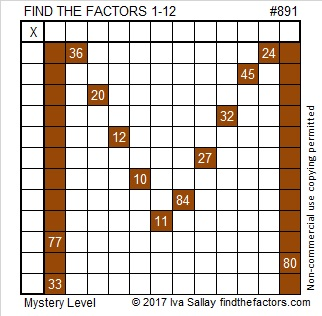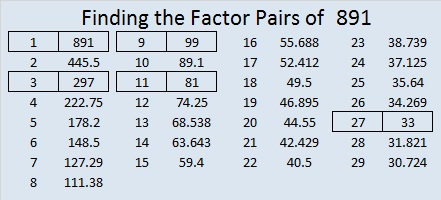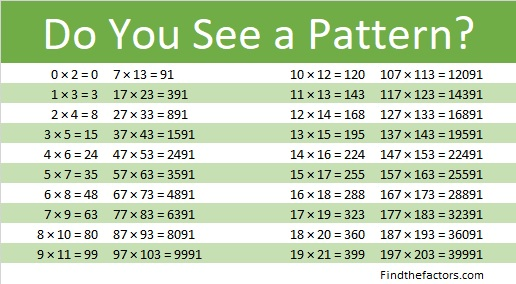# 891 Mystery Level Puzzle

Sometimes revealing the puzzle level reveals more than is needed. I think I will periodically publish a Mystery Level puzzle. Can you solve this one?Print the puzzles or type the solution on this excel file: 12 factors 886-896

8 + 9 + 1 = 18, so 891 is divisible by 3 and by 9.

8 – 9 + 1 = 0, so 891 is divisible by 11.

891 looks interesting in a few different bases:

• 1(2⁹) + 1(2⁸) + 0(2⁷) + 1(2⁶) + 1(2⁵) + 1(2⁴) + 1(2³) + 0(2²) + 1(2¹) + 1(2⁰) =891, so it’s palindrome 1101111011 in BASE 2.
• RR in BASE 32 (R is 27 in base 10), because 27(32) + 27(1) = 27(33) = 891
• R0 in BASE 33, because 27(33) = 891

891 is also the sum of five consecutive prime numbers: 167 + 173 + 179 + 181 + 191 = 891

• 891 is a composite number.
• Prime factorization: 891 = 3 × 3 × 3 × 3 × 11, which can be written 891 = 3⁴ × 11
• The exponents in the prime factorization are 4 and 1. Adding one to each and multiplying we get (4 + 1)(1 + 1) = 5 × 2 = 10. Therefore 891 has exactly 10 factors.
• Factors of 891: 1, 3, 9, 11, 27, 33, 81, 99, 297, 891
• Factor pairs: 891 = 1 × 891, 3 × 297, 9 × 99, 11 × 81, or 27 × 33
• Taking the factor pair with the largest square number factor, we get √891 = (√81)(√11) = 9√11 ≈ 29.849623891 is in this cool pattern: